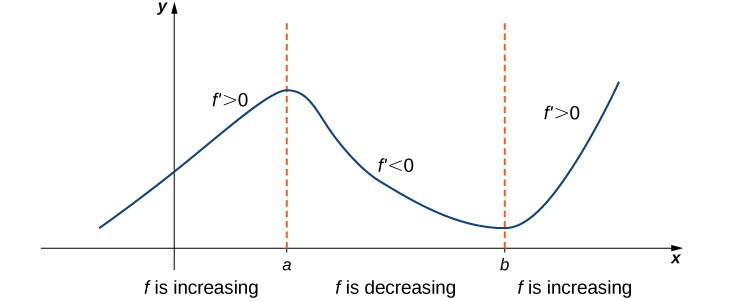# 4.4 The mean value theorem  (Page 4/7)

 Page 4 / 7
$\frac{f\left(b\right)-f\left(a\right)}{b-a}\ne 0.$

Since $f$ is a differentiable function, by the Mean Value Theorem, there exists $c\in \left(a,b\right)$ such that

${f}^{\prime }\left(c\right)=\frac{f\left(b\right)-f\left(a\right)}{b-a}.$

Therefore, there exists $c\in I$ such that ${f}^{\prime }\left(c\right)\ne 0,$ which contradicts the assumption that ${f}^{\prime }\left(x\right)=0$ for all $x\in I.$

From [link] , it follows that if two functions have the same derivative, they differ by, at most, a constant.

## Corollary 2: constant difference theorem

If $f$ and $g$ are differentiable over an interval $I$ and ${f}^{\prime }\left(x\right)={g}^{\prime }\left(x\right)$ for all $x\in I,$ then $f\left(x\right)=g\left(x\right)+C$ for some constant $C.$

## Proof

Let $h\left(x\right)=f\left(x\right)-g\left(x\right).$ Then, ${h}^{\prime }\left(x\right)={f}^{\prime }\left(x\right)-{g}^{\prime }\left(x\right)=0$ for all $x\in I.$ By Corollary 1, there is a constant $C$ such that $h\left(x\right)=C$ for all $x\in I.$ Therefore, $f\left(x\right)=g\left(x\right)+C$ for all $x\in I.$

The third corollary of the Mean Value Theorem discusses when a function is increasing and when it is decreasing. Recall that a function $f$ is increasing over $I$ if $f\left({x}_{1}\right) whenever ${x}_{1}<{x}_{2},$ whereas $f$ is decreasing over $I$ if $f{\left(x\right)}_{1}>f\left({x}_{2}\right)$ whenever ${x}_{1}<{x}_{2}.$ Using the Mean Value Theorem, we can show that if the derivative of a function is positive, then the function is increasing; if the derivative is negative, then the function is decreasing ( [link] ). We make use of this fact in the next section, where we show how to use the derivative of a function to locate local maximum and minimum values of the function, and how to determine the shape of the graph.

This fact is important because it means that for a given function $f,$ if there exists a function $F$ such that ${F}^{\prime }\left(x\right)=f\left(x\right);$ then, the only other functions that have a derivative equal to $f$ are $F\left(x\right)+C$ for some constant $C.$ We discuss this result in more detail later in the chapter.If a function has a positive derivative over some interval I , then the function increases over that interval I ; if the derivative is negative over some interval I , then the function decreases over that interval I .

## Corollary 3: increasing and decreasing functions

Let $f$ be continuous over the closed interval $\left[a,b\right]$ and differentiable over the open interval $\left(a,b\right).$

1. If ${f}^{\prime }\left(x\right)>0$ for all $x\in \left(a,b\right),$ then $f$ is an increasing function over $\left[a,b\right].$
2. If ${f}^{\prime }\left(x\right)<0$ for all $x\in \left(a,b\right),$ then $f$ is a decreasing function over $\left[a,b\right].$

## Proof

We will prove i.; the proof of ii. is similar. Suppose $f$ is not an increasing function on $I.$ Then there exist $a$ and $b$ in $I$ such that $a but $f\left(a\right)\ge f\left(b\right).$ Since $f$ is a differentiable function over $I,$ by the Mean Value Theorem there exists $c\in \left(a,b\right)$ such that

${f}^{\prime }\left(c\right)=\frac{f\left(b\right)-f\left(a\right)}{b-a}.$

Since $f\left(a\right)\ge f\left(b\right),$ we know that $f\left(b\right)-f\left(a\right)\le 0.$ Also, $a tells us that $b-a>0.$ We conclude that

${f}^{\prime }\left(c\right)=\frac{f\left(b\right)-f\left(a\right)}{b-a}\le 0.$

However, ${f}^{\prime }\left(x\right)>0$ for all $x\in I.$ This is a contradiction, and therefore $f$ must be an increasing function over $I.$

## Key concepts

• If $f$ is continuous over $\left[a,b\right]$ and differentiable over $\left(a,b\right)$ and $f\left(a\right)=0=f\left(b\right),$ then there exists a point $c\in \left(a,b\right)$ such that ${f}^{\prime }\left(c\right)=0.$ This is Rolle’s theorem.
• If $f$ is continuous over $\left[a,b\right]$ and differentiable over $\left(a,b\right),$ then there exists a point $c\in \left(a,b\right)$ such that
$f\prime \left(c\right)=\frac{f\left(b\right)-f\left(a\right)}{b-a}.$

This is the Mean Value Theorem.
• If $f\prime \left(x\right)=0$ over an interval $I,$ then $f$ is constant over $I.$
• If two differentiable functions $f$ and $g$ satisfy ${f}^{\prime }\left(x\right)={g}^{\prime }\left(x\right)$ over $I,$ then $f\left(x\right)=g\left(x\right)+C$ for some constant $C.$
• If ${f}^{\prime }\left(x\right)>0$ over an interval $I,$ then $f$ is increasing over $I.$ If ${f}^{\prime }\left(x\right)<0$ over $I,$ then $f$ is decreasing over $I.$

How to do basic integrals
write something lmit
find the integral of tan tanxdx
-ln|cosx| + C
Jug
discuss continuity of x-[x] at [ _1 1]
Given that u = tan–¹(y/x), show that d²u/dx² + d²u/dy²=0
find the limiting value of 5n-3÷2n-7
Use the first principal to solve the following questions 5x-1
175000/9*100-100+164294/9*100-100*4
mode of (x+4) is equal to 10..graph it how?
66
ram
6
ram
6
Cajab
what is domain in calculus
nelson
integrals of 1/6-6x-5x²
derivative of (-x^3+1)%x^2
(-x^5+x^2)/100
(-5x^4+2x)/100
oh sorry it's (-x^3+1)÷x^2
Misha
-5x^4+2x
sorry I didn't understan A with that symbol
find the derivative of the following y=4^e5x y=Cos^2 y=x^inx , x>0 y= 1+x^2/1-x^2 y=Sin ^2 3x + Cos^2 3x please guys I need answer and solutions
differentiate y=(3x-2)^2(2x^2+5) and simplify the result
Ga
72x³-72x²+106x-60
okhiria
y= (2x^2+5)(3x+9)^2
lemmor
solve for dy/dx of y= 8x^3+5x^2-x+5
192x^2+50x-1
Daniel
are you sure? my answer is 24x^2+10x-1 but I'm not sure about my answer .. what do you think?
Ga
24x²+10x-1
Ga
yes
ok ok hehe thanks nice dp ekko hahaha
Ga
hahaha 😂❤️❤️❤️ welcome bro ❤️
Ga
y= (2x^2+5)(3x+9)^2
lemmor
can i join?
Fernando
yes of course
Jug
can anyone teach me integral calculus?
Jug
it's just the opposite of differential calculus
yhin
of coursr
okhiria
but i think, it's more complicated than calculus 1
Jug
Hello can someone help me with calculus one...
Jainaba
find the derivative of y= (2x+3)raise to 2 sorry I didn't know how to put the raise correctly
8x+12
Dhruv
8x+3
okhiria
d the derivative of y= e raised to power x
okhiria
rates of change and tangents to curves
how can find differential Calculus
KyawByBy Lakeima RobertsBy Edward BitonByByByBy